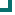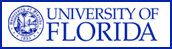Enterococcus faecium (NORTHCENTRAL Data) 2004 2005 2006 2007 2008 2009 2010 ampicillin 23.2%n=56 10.9%n=101 12.2%n=82 10.6%n=104 16.7%n=42 10.6%n=141 vancomycin 63.5%n=156 47.5%n=101 36.6%n=82 32.4%n=102 35.7%n=42 21.3%n=141 quinupristin/dalfopristin 100%n=17 100%n=11 91.7%n=12 94.1%n=17 nitrofurantoin 94.1%n=17 100%n=11 10%n=30 9.7%n=124 tetracycline 58.8%n=17 27.3%n=11 42.9%n=35 83.3%n=12 64.7%n=17 gentamicin 41.2%n=17 54.5%n=11 rifampim 52.9%n=17 54.5%n=11 Totals 57.6%n=297 37.4%n=257 24.4%n=164 24.5%n=241 33.3%n=138 19.1%n=440

This data is accurate as of: 11/22/2016 11:53:45 AM

Drugs with less than 10 isolates are removed from the chart and from the graph. The following drugs had some data removed: ampicillin, quinupristin/dalfopristin, nitrofurantoin, tetracycline, gentamicin, rifampim, vancomycin.ARMProgram.com is a project run by the University of Florida. Copyright 2011. All Rights Reserved. Site by JMarc Technologies, LLC